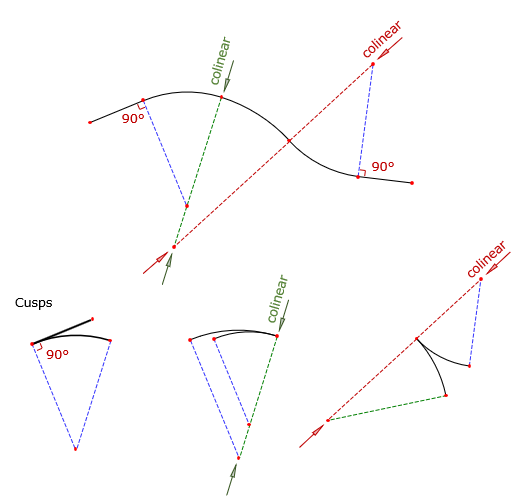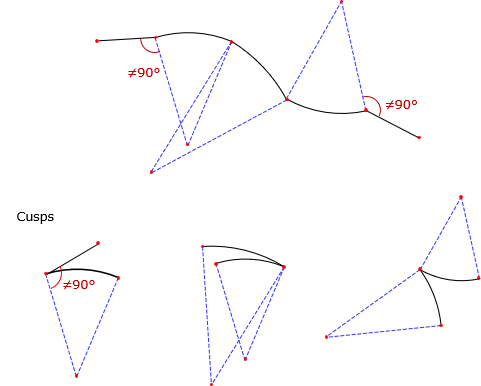### 3. Tangency Condition

At its ends, an arc can be joined to a straight line or another arc. At the connection point, the relationship with the adjacent boundary can be tangent or non tangent.

For a tangent condition, Figure G-6:

• Line to arc (and arc to line) - line is perpendicular to the radius at the intersection.
• Arc to Arc - intersection point and radius point of both arcs are collinear; all three point are on a straight line.Figure G-6 Tangent conditions

Any deviation from those constraints results in a non-tangent transition, Figure G-7.Figure G-7 Non-tangent conditions

Tangent transitions (except for cusps) are particularly important in transportation network design. A tangent condition provides a smooth directional transition from one segment to another, important for passenger safety and vehicle dynamics. Non-tangent conditions are generally limited to controlled intersections where the transporting vehicle is brought to a complete stop before changing direction.

A typical subdivision will have a mix of tangent and non-tangent curves. For example, streets are designed using tangent curves while lot lines intersecting streets usually do so in non-tangent conditions, Figure G-8.Figure G-8 Tangent and non-tangent conditions